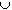#Interactive Real Analysis

Next | Previous | Glossary | Map

## 7.3. Measures

### Examples 7.3.10(a): Properties of Measure

Show that for any two sets A and B we have that
m(A - B) = m(A) - m(AB)
What if BA?
For any sets A and B we have:
(AB)(Acomp(B)) = A
and AB and Acomp(B) are disjoint. Therefore, by additivity of measure we have:
m(AB) + m(Acomp(B)) = m(A)
Therefore
m(A - B) = m(A) - m(AB)
as we had to prove. If BA then AB = B so that
m(A - B) = m(A) - m(B)
Next | Previous | Glossary | Map